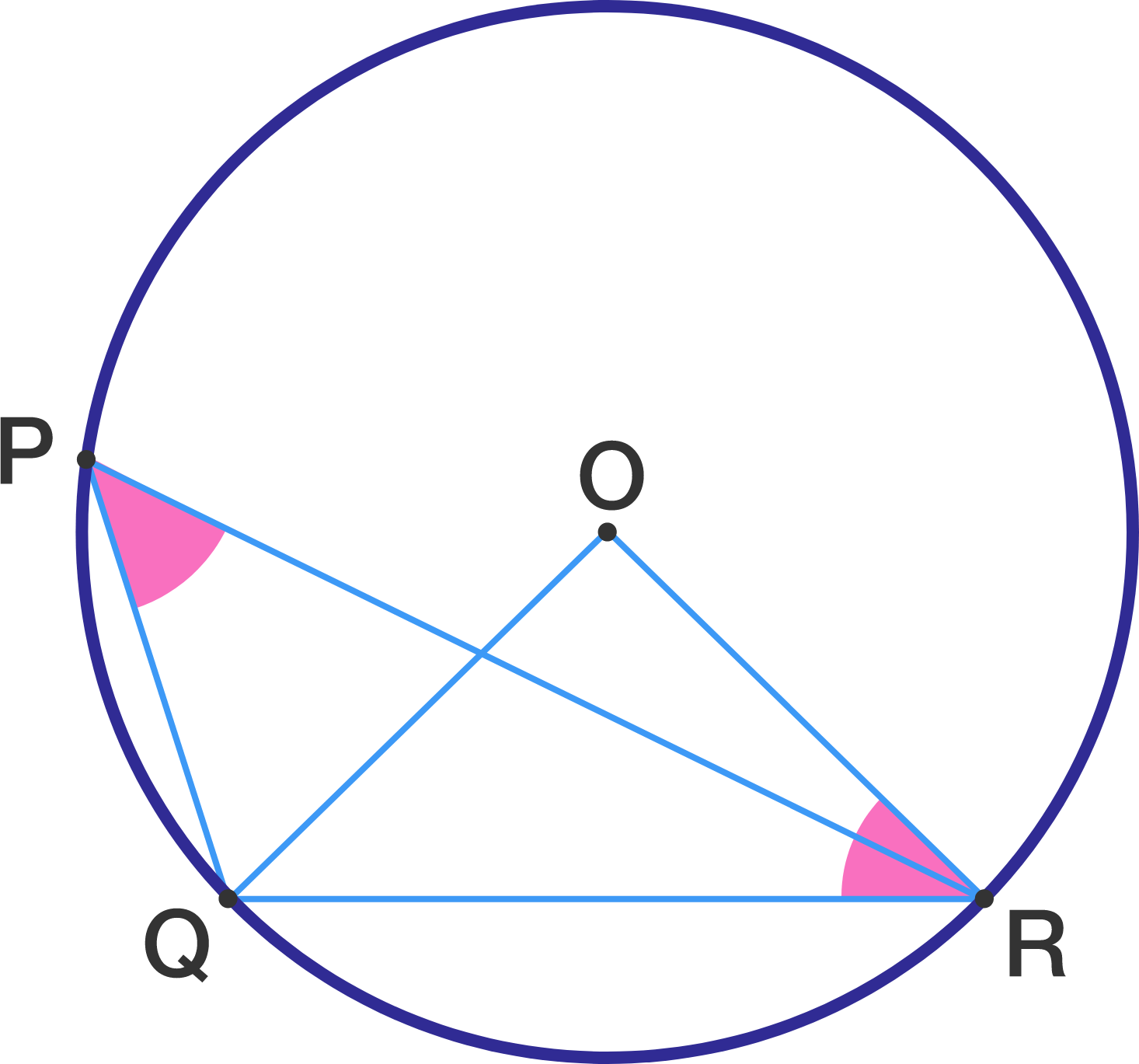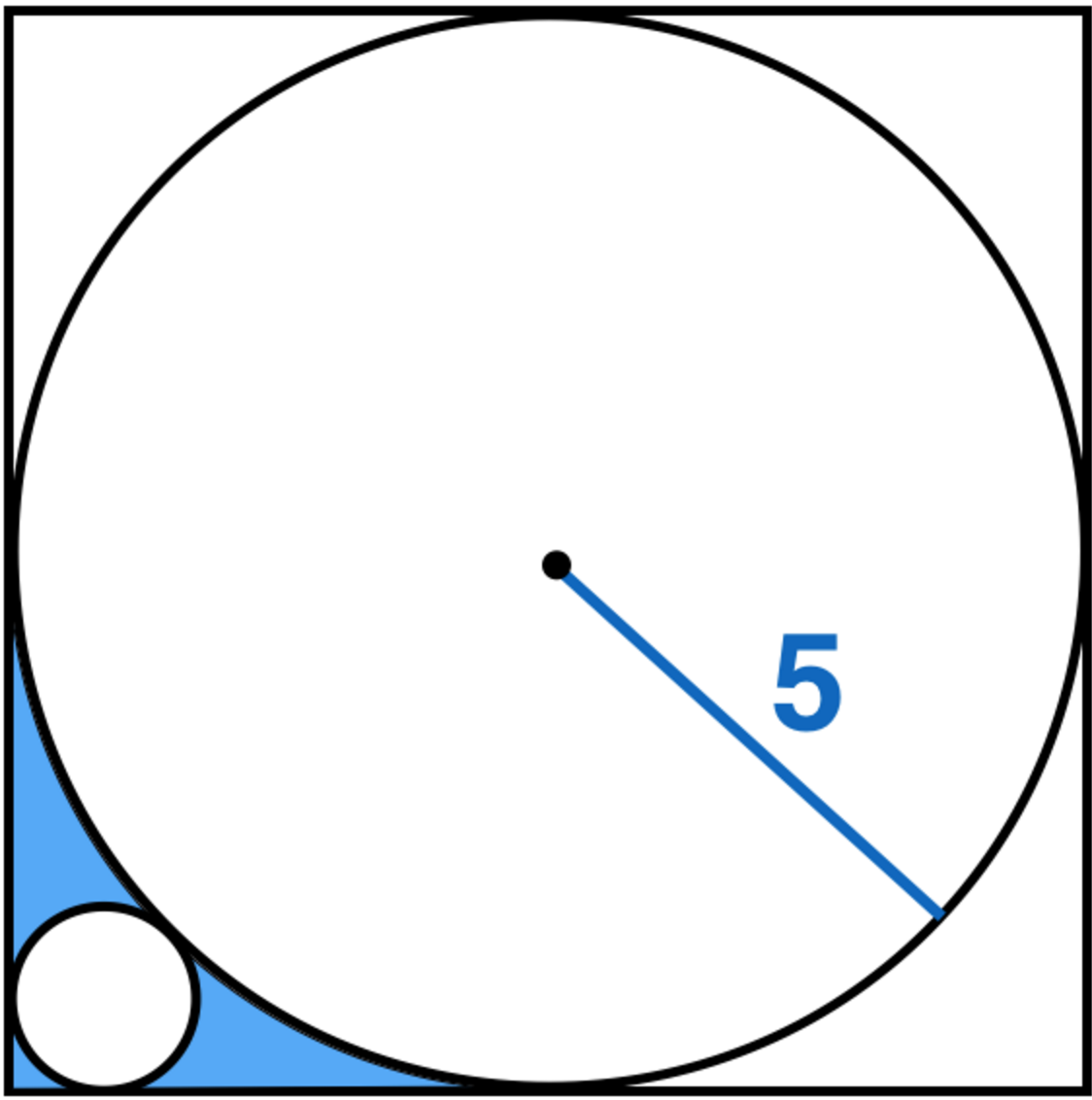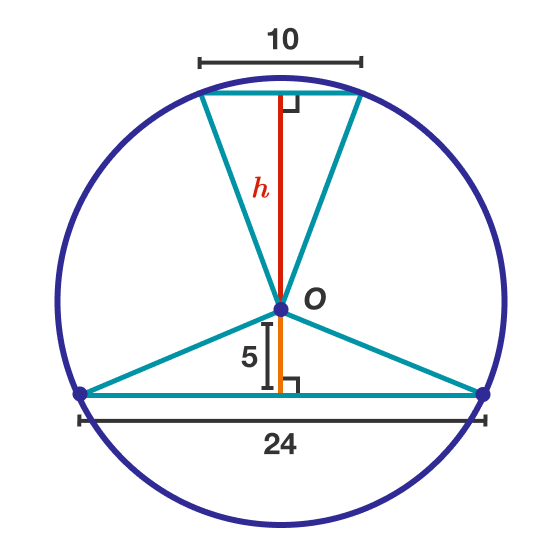Geometry

# Circle Properties: Level 2 ChallengesThree semicircles (with equal radii) are drawn inside the large semicircle so that their diameters all sit on the diameter of the large semicircle. What is the ratio of the red area to the blue area?$O$ is the center of the circle to the right.

Find, in degrees, $\angle QPR + \angle ORQ.$A circle is inscribed in a square as shown above. A smaller circle is drawn tangent to two sides of the square and externally tangent to the inscribed circle. Find the area of the blue shaded region to two decimal places.Five congruent circles overlap. A line is drawn connecting the bottom of the first circle to the top of the fifth circle. The area enclosed by the circles under the line is shaded gold.

The overlapping areas of two circles each have an area of 5, and the gold area is 35.

Find the area of one circle.$O$ is the center of the circle. Find $h.$

×